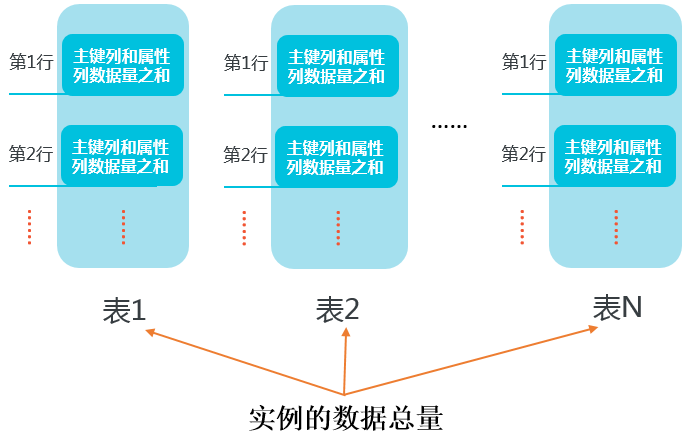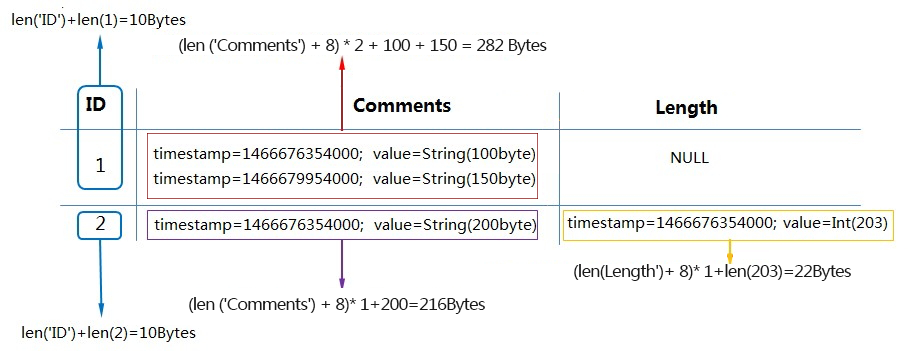## 计算行数据量

• 主键列的数据量 = 主键列的名字长度之和 + 主键列的值的数据量之和

• 属性列的数据量计算方式，请参考本文档中关于行及表的数据量计算示例的具体说明。

String UTF-8字符串占用的字节数（表格存储允许值为空的String类型，如果字符串为空，则数据大小为0。）
Integer 8
Double 8
Boolean 1
Binary 二进制数据占用的字节数

ID Name Length Comments
1 timestamp = 1466676354000, value = ‘zhangsan’ timestamp = 1466676354000, value = 20

timestamp = 1466676354000, value = String （100 Bytes）

timestamp = 1466679954000, value = String （150 Bytes）

• 当MaxVersions = 2，TTL = 2592000时：
单个属性列的数据量 = （属性列名字长度之和 + 8） * 有效版本个数 + 该属性列所有有效版本的值数据量之和
说明 在使用多版本（即Max versions > 1）或者使用了`TTL（即 TTL!= -1）`的情况下，每个版本号需要占用8字节（以下提到的timestamp等同于版本号）。
该行数据量 = 10 + 20 + 22 + 282 = 334 Bytes，详情如下：
• 主键列数据量 = `len (‘ID’) + len (1) = 10 Bytes`
• 属性列 Name 数据量 = `(len (‘Name’) + 8) * 1 + len (‘zhangsan’) = 20 Bytes`
• 属性列 Length 数据量 = `(len (‘Length’) + 8) * 1 + len (20) = 22 Bytes`
• 属性列 Comments 数据量 = `(len (‘Comments’) + 8) * 2 + 100 + 150 = 282 Bytes`
• 当MaxVersions = 1，TTL = -1时：

单个属性列的数据量 = 属性列名字长度之和 + 属性列的值的数据量之和

说明
• 在不使用多版本（即Max versions = 1）且不使用TTL（即TTL = -1）的场景下，版本号不占用字节。
• 虽然Comments有两个版本，但由于MaxVersions = 1，只计算最新的版本。

该行数据量 = 10 + 12 + 14 + 158 = 194 Bytes，详情如下：

• 主键列数据量 = `len (‘ID’) + len (1) = 10 Bytes`
• 属性列Name数据量 = `len (‘Name’) + len (‘zhangsan’) = 12 Bytes`
• 属性列Length数据量 = `len (‘Length’) + len (20) = 14 Bytes`
• 属性列Comments数据量 = `len (‘Comments’) + 150 (Bytes) = 158 Bytes`

## 计算表数据量• 对于ID = 1的行，其数据量 = 10（主键列数据量）+282（Comments属性列两个版本的数据量） = 292 Bytes。
• 对于ID = 2的行，其数据量 = 10（主键列数据量）+ 216（Comments属性列一个版本的数据量）+ 22（Length属性列一个版本的数据量）= 248 Bytes。
• 因此该表的数据量之和为292 + 248 = 540 Bytes。